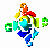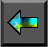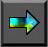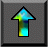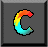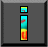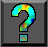Next: Chapter Notes Up: 1 Parallel Computers and Computation Previous: 1.5 Summary

# Exercises

Exercises 6--10 require you to describe a parallel algorithm. You should describe the task/channel structure created by the algorithm and provide a definition for each task, including its interface (inports and outports), its local data, and the actions it performs.

1. If today's workstations execute at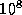operations per second, and performance increases at a rate of 25 percent per year, how long will it be before we have workstations capable of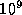operations per second?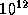?

2. A climate model requires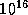floating point operations for a ten-year simulation. How long would this computation take at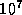floating point operations per second (10 Mflops)?

3. A climate model generates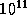bytes of data in a ten-day simulation. How fast must data be transferred to secondary storage? What transfer rate is required if we are to search this data in ten minutes?

4. Consider a three-dimensional chip. Demonstrate that chip volume V and computation time T are related as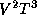, just as area A and computation time are related as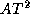in a two-dimensional chip.

5. Execute the parallel algorithm described in Section 1.4.1 by hand for N=4 , and satisfy yourself that execution is deterministic.

6.   Adapt the parallel algorithm of Section 1.4.1 to deal with a two-dimensional finite difference problem in which the value of each point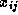in a two-dimensional grid of size N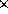N is updated as follows: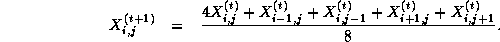7. Describe a variant of the parallel algorithm of Section 1.4.2 that allows for the case when N is even.

8. Describe a parallel algorithm for Hoare's quicksort algorithm  based on the parallel divide-and-conquer strategy employed in Section 1.4.3.

9. Describe a task/channel structure for a parallel database system in which M concurrently executing users can generate requests to read and write data located in N databases and requests to access different databases can be handled concurrently. You must use less than M.N channels.

Extend this structure to allow a user to request that a set of read and write operations be performed as an atomic operation, that is, without read or write operations generated by other tasks intervening.

10.   Extend the parallel algorithms of Sections 1.4.1 and 1.4.3 to provide for the loading of initial data values in from disk and the writing out of the solutions to disk.Next: Chapter Notes Up: 1 Parallel Computers and Computation Previous: 1.5 Summary